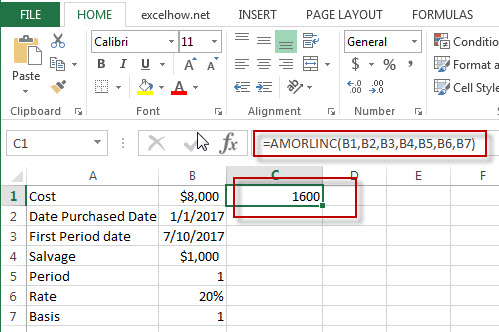## Excel Amorlinc Function

This post will guide you how to use Excel AMORLINC function with syntax and examples in Microsoft excel.

### Description

The Excel AMORLINC function returns the linear depreciation of an asset for each accounting period. This function is provided for French accounting system.

The AMORLINC function is a build-in function in Microsoft Excel and it is categorized as a Financial Function.

The AMORLINC function is available in Excel 2016, Excel 2013, Excel 2010, Excel 2007, Excel 2011 for Mac.

### Syntax

The syntax of the AMORLINC function is as below:

`= AMORLINC(cost, date_purchased, first_period, salvage, period, rate, [basis])`

Where the AMORLINC function arguments are:

• Cost -This is a required argument. The cost of the asset.
• Date_purchased – This is a required argument.  The date that the asset was purchased.
• First_period – This is a required argument.  The date of the end of the first period.
• Salvage – This is a required argument.  The salvage value at the end of the life of the asset.
• Period – This is a required argument.  The period in which to calculate the depreciation.
• Rate– This is a required argument.  The rate of depreciation.
• Basis – This is a required argument.  The year basis to be used. The following values can be used:
 Basis Date system 0 or omitted 360 days (NASD method) 1 Actual 3 365 days in a year 4 360 days in a year (European method)

### Excel AMORLINC  Function Example

The below examples will show you how to use Excel AMORLINC Function to calculate the linear depreciation of an asset for each accounting period.

#1 = AMORLINC (B1,B2,B3,B4,B5,B6,B7)### Related Functions

• Excel Amordegrc Function
The Excel AMORDEGRC function returns the linear depreciation of an asset for each accounting period by using a depreciation coefficient.The syntax of the AMORDEGRC function is as below:= AMORDEGRC (cost, date_purchased, first_period, salvage, period, rate, [basis])…